Click in-app to access the full platform documentation for your version of DataRobot.

# ROC Curve tools¶

The ROC Curve tab provides tools for exploring classification, performance, and statistics related to a selected model at any point on the probability scale. Because choosing the best model is based on a number of parameters, it is important to understand whether the classification performance of a particular model meets your specifications.

## ROC Curve tab components¶

To access the ROC Curve, navigate to the Leaderboard, select the model you want to evaluate, then click Evaluate > ROC Curve. The ROC Curve tab contains the set of interactive graphical displays described below.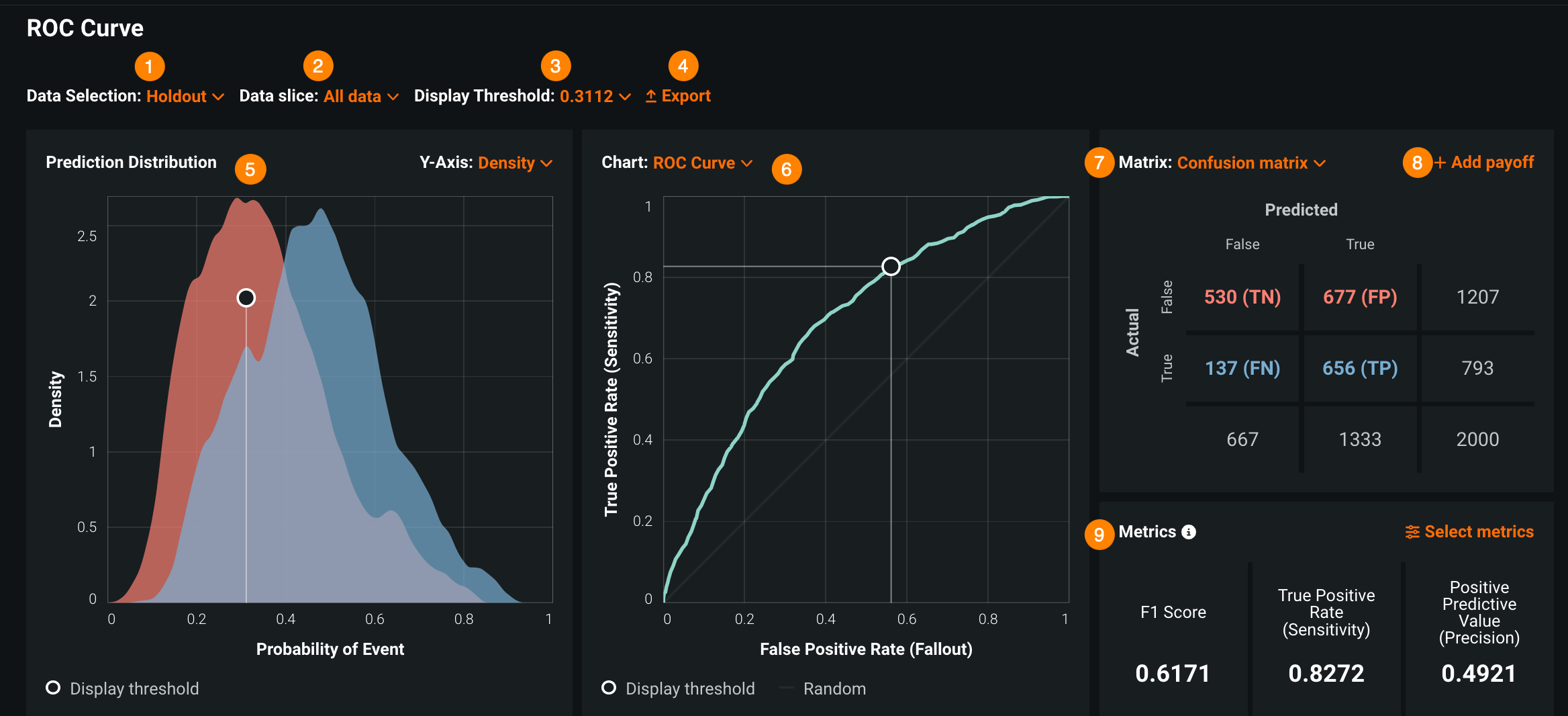Element DescriptionData Selection Select the data source for your visualization. The options available—Holdout, Cross Validation, and Validation—are dependent on whether you have run or enabled that partition. You can also choose to Add external test data. This link takes you to the Predict > Make Predictions tab where you can add test data and run an external test. Then return to the ROC Curve tab, click Data Selection, and select the test you ran.Display Threshold Select a display threshold that separates predictions classified as "false" from predictions classified as "true."Export Export to a CSV, PNG, or ZIP file:
• Download a PNG of a ROC Curve, Profit Curve, Prediction Distribution graph, Cumulative Gain chart, or a Cumulative Lift chart.
• Download a ZIP file containing all of the CSV and PNG files.Prediction Distribution Use the Prediction Distribution graph to evaluate how well your classification model discriminates between the positive and negative classes. The graph separates predictions classified as "true" from predictions classified as "false" based on the prediction threshold you set.Chart selector Select a type of chart to display. Choose from ROC Curve (default), Average Profit, Precision Recall, Cumulative Lift (Positive/Negative), and Cumulative Gain (Positive/Negative). You can also create your own custom chart.Matrix selector Select a type of matrix to display. By default, a confusion matrix displays. You can choose to display the confusion matrix data by instance counts or percentages. You can instead create a payoff matrix so that you can generate and view a profit curve.+ Add payoff Enter payoff values to generate a profit curve so that you can estimate the business impact of the model. Clicking Add payoff displays a Payoff Matrix in the Matrix pane if not already displayed. Adjust the Payoff values in the matrix and set the Chart pane to Average Profit to view the impact.Metrics View summary statistics that describe model performance at the selected threshold. You can use the Select metrics menu to choose up to three metrics to display at one time.

To use these components, select a threshold between predictions classified as "true" or "false"—each component works together to provide an interactive snapshot of the model's classification behavior based on that threshold.

Tip

Several Wikipedia pages and the Internet in general provide thorough descriptions explaining many of the elements provided by the ROC Curve tab. Some are summarized in the sections that follow.

## Classification use cases¶

The following sections use one of two binary classification use cases to illustrate the concepts described. In both cases, each row in the dataset represents a single patient, and the features (columns) contain descriptive variables about the patient's medical condition.

The ROC curve is a graphical means of illustrating classification performance for a model as the relevant performance statistics at all points on the probability scale change. To understand the reported statistics, you must understand the four possible outcomes of a classification problem; these outcomes are the basis of the confusion matrix.

### Classification use case 1¶

Use case 1 asks "Does a patient have diabetes?" This hypothetical dataset has both categorical and numeric values and describes whether a patient has diabetes. The target variable, `has_diabetes`, is a categorical value that describes whether the patient has the disease (`has_diabetes=1`) or does not have the disease (`has_diabetes=0`). Numeric and other categorical variables describe factors like blood pressure, payer code, number of procedures, days in hospital, and more. For use case 1:

Outcome Description
True positive (TP) A positive instance that the model correctly classifies as positive. For example, a diabetic patient correctly identified as diabetic.
False positive (FP) A negative instance that the model incorrectly classifies as positive. For example, a healthy patient incorrectly identified as diabetic.
True negative (TN) A negative instance that the model correctly classifies as negative. For example, a healthy patient correctly identified as healthy.
False negative (FN) A positive instance that the model incorrectly classifies as negative. For example, a diabetic patient incorrectly identified as healthy.

The following points provide some statistical reasoning behind using the outcomes:

• Correct predictions: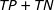• Incorrect predictions: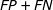• Total scored cases: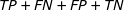• Error rate: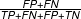• Overall accuracy (probability a prediction is correct):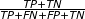### Classification use case 2¶

Use Case 2 is a model that tries to determine whether a diabetic patient will be readmitted to hospital (the target feature). This hypothetical dataset has both categorical and numeric values and describes whether a patient will be readmitted to the hospital within 30 days (target `variable=readmitted`). This categorical value describes whether the patient is readmitted inside of 30 days (`readmitted=1`) or is not readmitted within that time frame (`readmitted=0`); other categorical values include things like admission id and payer code. Numeric variables describe things like blood pressure, number of procedures, days in hospital, and more.

## ROC Curve tools¶

The ROC Curve tab provides the tools described in the following sections:

Tool Description
Confusion matrix Describes how to use a confusion matrix to evaluate model accuracy by comparing actual versus predicted values.
Display and prediction thresholds Shows how to use thresholds to set class boundaries in ROC Curve tab visualizations and model predictions.
Prediction Distribution graph Shows how to view the distribution of actual values in relation to the display threshold in the Prediction Distribution graph.
ROC curve Shows how to evaluate a module using a ROC curve visualization to plot the true positive rate against the false positive rate for a given data source.
Profit curve Explains how to generate profit curves that help you estimate the business impact of a selected model.
Cumulative charts Shows how to assess model performance by exploring the model's cumulative characteristics.
Custom charts Describes how to create custom charts that evaluate classification models.
Metrics summary Describes how to use the Metrics pane to explore statistics related to a selected model.

Note

DataRobot displays the ROC Curve tab only for models created for a binary classification target (a target with two unique values).

Updated November 21, 2021